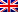#[Internetwache CTF 2016] [Reverse 80 – Eso Tape] Write Up

## Description

I once took a nap on my keyboard. I dreamed of a brand new language, but I could not decipher it nor get its meaning. Can you help me? Hint: Replace the spaces with either ‘{‘ or ‘}’ in the solution.

## Resolution

We found that it was the TapeBagel esoteric language.

Helped with the commands and operators, we managed to follow the execution process:

```##     ; resets all of the integers to zero                              //int0, int1, int2 = 0
%%     ; resets the integer index to zero                                //index = int0
%++    ; adds one to the integer that the integer index is pointing to   //int0 = 1
%++    ; adds one to the integer that the integer index is pointing to   //int0 = 2
%++    ; adds one to the integer that the integer index is pointing to   //int0 = 3
%#     ; adds one to the integer index                                   //index = int1
*&*    ; int0 * int0                                                     //int1 = 3 * 3 = 9
@**    ; outputs int1 as a character (1 = A, 2 = B, ... 26 = Z)          //output 9 = I

%#     ; adds one to the integer index                                   //index = int2
**&*   ; int1 * int0                                                     //int2 = 9 * 3 = 27
***-*  ; int2 - int0                                                     //int2 = 27 - 3 = 24
***-*  ; int2 - int0                                                     //int2 = 24 - 3 = 21
%++    ; adds one to the integer that the integer index is pointing to   //int2 = 22
%++    ; adds one to the integer that the integer index is pointing to   //int2 = 23
@***   ; ouputs int2                                                     //output 23 = W

*-*    ; int0 - int0                                                     //int2 = 3 - 3 = 0
@***   ; outputs int2                                                    //output 0 = NULL = {

@**    ; outputs int1                                                    //output 9 = I

*+**   ; int0 + int1                                                     //int2 = 3 + 9 = 12
@***   ; outputs int2                                                    //output 12 = L

***+*  ; int2 + int0                                                     //int2 = 12 + 3 = 15
@***   ; outputs int2                                                    //output 15 = O
**+**  ; int1 + int1                                                     //int2 = 9 + 9 = 18
***+*  ; int2 + int0                                                     //int2 = 18 + 3 = 21
%++    ; adds one to the integer that the integer index is pointing to   //int2 = 22
@***   ; outputs int2                                                    //output 22 = V
#%     ; sets all of the integers to one                                 //int0, int1, int2 = 1
%%     ; resets the integer index to zero                                //index = int0
%++    ; adds one to the integer that the integer index is pointing to   //int0 = 2
%++    ; adds one to the integer that the integer index is pointing to   //int0 = 3
%++    ; adds one to the integer that the integer index is pointing to   //int0 = 4
%++    ; adds one to the integer that the integer index is pointing to   //int0 = 5
@*     ; outputs int0                                                    //output 5 = E

%#     ; adds one to the integer index                                   //index = int1
%++    ; adds one to the integer that the integer index is pointing to   //int1 = 2
%++    ; adds one to the integer that the integer index is pointing to   //int1 = 3
%++    ; adds one to the integer that the integer index is pointing to   //int1 = 4
%%     ; resets the integer index to zero                                //index = int0
*&**   ; int0 * int1                                                     //int0 = 5 * 4 = 20
@*     ; outputs int0                                                    //output 20 = T

@***   ; outputs int2                                                    //output 1 = A

*-**   ; int0 - int1                                                     //int0 = 20 - 4 = 16
@*     ; outputs int0                                                    //output 16 = P

%#     ; adds one to the integer index                                   //index = int1
%++    ; adds one to the integer that the integer index is pointing to   //int1 = 5
@**    ; outputs int1                                                    //output 5 = E

*-**   ; int0 - int1
*-**   ; int0 - int1
**-*** ; int1 - int2
**-*** ; int1 - int2
**-*** ; int1 - int2
@**    ; outputs int1                                                    //output 2 = B

@***   ; outputs int2                                                    //output 1 = A

#%     ; sets all of the integers to one
%%     ; resets the integer index to zero
%++    ; adds one to the integer that the integer index is pointing to
%++    ; adds one to the integer that the integer index is pointing to
%++    ; adds one to the integer that the integer index is pointing to
%++    ; adds one to the integer that the integer index is pointing to
%#     ; adds one to the integer index
*+**   ; int0 + int1
%++    ; adds one to the integer that the integer index is pointing to
@**    ; outputs int1                                                    //output 7 = G

@*     ; outputs int0                                                    //output 5 = E

%#     ; adds one to the integer index
*+**   ; int0 + int1
@***   ; outputs int2                                                    //output 12 = L

##     ; resets all of the integers to zero
%%     ; resets the integer index to zero
@***   ; outputs int2                                                    //output NULL = }
```

Flag was : IW{ILOVETAPEBAGEL}